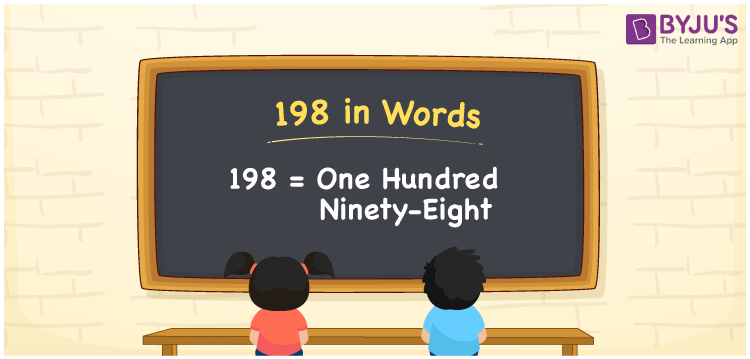# 198 in words

198 in words is written as One Hundred and Ninety Eight. 198 represents the count or value. The article on Counting Numbers can give you an idea about count or counting. The number 198 is a 3 digit number that is used in expressions related to money, days, distance, length, weight and so on. Let us consider an example for 198. “There are One Hundred and Ninety Eight movies stored in my hard disk.”

 198 in words One Hundred and Ninety Eight One Hundred and Ninety Eight in Numbers 198

## 198 in English Words## How to Write 198 in Words?

We can convert 198 to words using a place value chart. The number 198 has 3 digits, so let’s make a chart that shows the place value up to 3 digits.

 Hundreds Tens Ones 1 9 8

Thus, we can write the expanded form as:

1 × Hundred + 9 × Ten + 8 × One

= 1 × 100 + 9 × 10 + 8 × 1

= 198

= One Hundred and Ninety Eight.

198 is the natural number that is succeeded by 197 and preceded by 199.

198 in words – One Hundred and Ninety Eight.

Is 198 an odd number? – No.

Is 198 an even number? – Yes.

Is 198 a perfect square number? – No.

Is 198 a perfect cube number? – No.

Is 198 a prime number? – No.

Is 198 a composite number? – Yes.

## Solved Example

1. Write the number 198 in expanded form

Solution: 1 × 100 + 9 × 10 + 8 × 1

We can write 198 = 100 + 90 + 8

= 1 × 100 + 9 × 10 + 8 × 1.

## Frequently Asked Questions on 198 in words

Q1

### How to write the number 198 in words?

198 in words is written as One Hundred and Ninety Eight.
Q2

### Is 198 divisible by 3?

Yes. 198 is divisible by 3.
Q3

### Is 198 a perfect square number?

No. 198 is not a perfect square number.